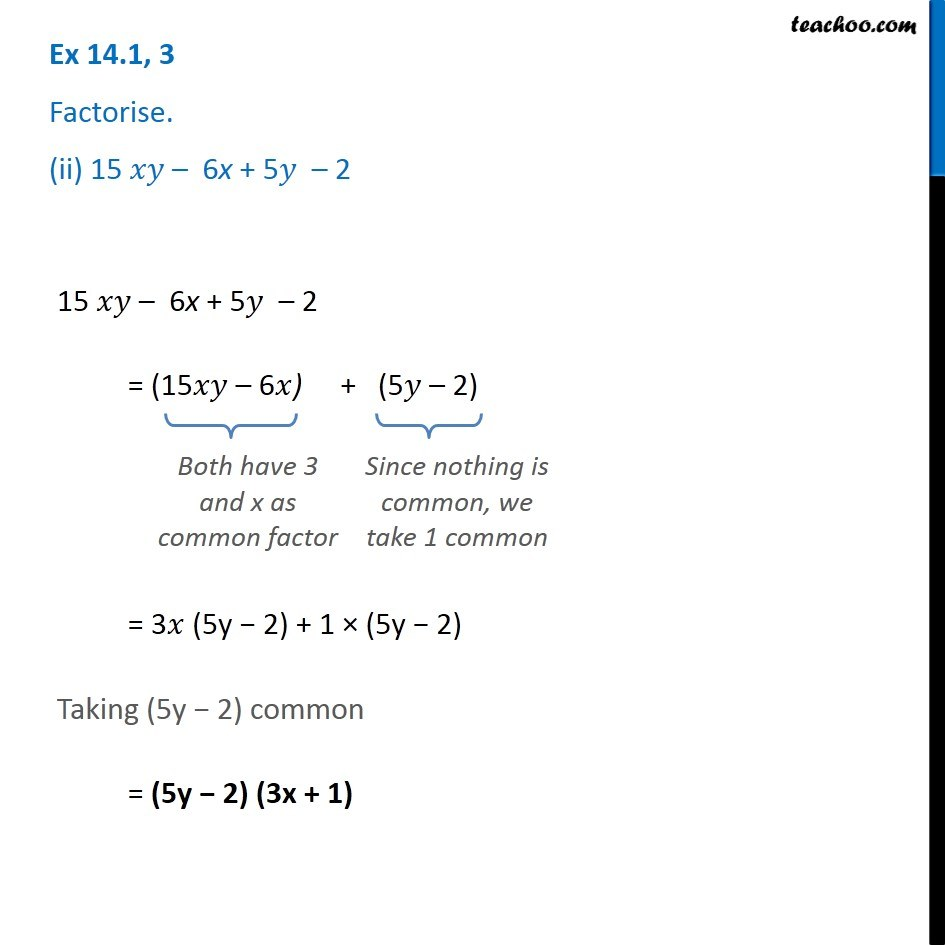Ex 14.1

Chapter 14 Class 8 Factorisation
Serial order wiseGet live Maths 1-on-1 Classs - Class 6 to 12

### Transcript

Ex 14.1, 3 Factorise. (ii) 15 𝑥𝑦 – 6x + 5𝑦 – 2 15 𝑥𝑦 – 6x + 5𝑦 – 2 = (15𝑥𝑦 – 6𝑥) + (5𝑦 – 2) Both have 3 and x as common factor Since nothing is common, we take 1 common = 3𝑥 (5y − 2) + 1 × (5y − 2) Taking (5y − 2) common = (5y − 2) (3x + 1)